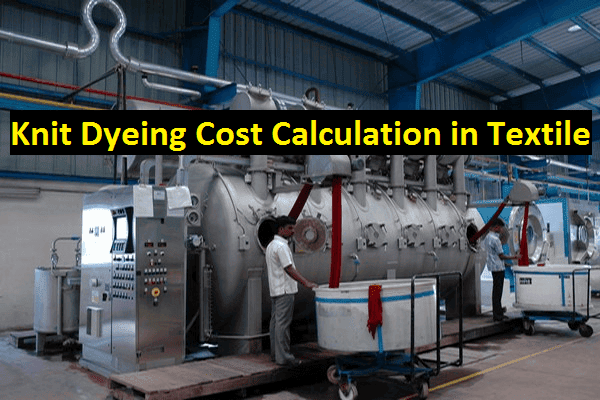### How to Calculate Knit Dyeing Cost in Textile Wet Processing Industry?

Profit or Loss Calculation of Knit Dyeing Project:
A textile engineer who serves his duty in wet processing industry has to know the actual method of knit dyeing calculation with its cost analysis. Otherwise he cannot perform his best in this sector. As its importance, this article has presented an easy method for calculation knit dyeing production with its cost analysis.Knit dyeing cost calculation in textile
Knit Dyeing Cost Calculation and Cost Analysis in Textile:
In this article, we calculate and analysis of a knit dyeing project (5 ton per day capacity) about its profit or loss percentage per day by using three steps.

Let,
5 tons knit fabrics will be dyed with the same recipe where sales per kg of dyeing that means dyeing charge is 105 taka.

a. Dyeing Recipe and Reagent Cost:

M: L= 1:8,
Thus, liquor required here= (8×5000) = 40000 liter.

 SL No. Reagent Recipe amount Reagent amount for 5 tons (in kg) Unit costs of reagent (tk/kg) Cost for reagent in tk 01 Wetting agent 0.5 gm/l {(40000×0.5)/1000}=20 200 8000 02 Sequestering agent 2.0 gm/l {(40000×2)/1000}=80 300 24000 03 Stabilizer 1.0 gm/l {(40000×1)/1000}=40 300 12000 04 Caustic soda 3.0 gm/l {(40000×3)/1000}=120 100 12000 05 H peroxide 4.0 gm/l {(40000×4)/1000}=160 50 8000 06 Levafix blue CA 0.25% {(5000×0.25)/100}=12.5 1400 17500 07 Levafix yellow CA 2.80% {(5000×2.80)/100}=140 500 70000 08 Levafix red CA 0.85% {(5000×0.85)/100}=42.5 400 17000 09 Salt 70 gm/l {(40000×70)/1000}=2800 10 28000 10 Soda ash 20 gm/l {(40000×20)/1000}=800 150 120000 11 Soaping agent 2.0 gm/l {(40000×2)/1000}=80 300 24000 12 Softener 1.00% {(5000×1.00)/100}=50 250 12500 Total 353000
b.  Miscellaneous Cost:
 SL No. Purpose Cost per kg dyeing Total cost for 5 tons 01 Salary 20 100000 02 Utilities 8 40000 03 Bank interest 0.1 500 04 Others 2 10000 Total 30.1 150500

c.  Profit or Loss Calculation:

Now,
Finally, we have to calculate here the profit or loss for this project.

As we know,
Sales per kg of dyeing is 105 taka,

So,
Total income from sale is 525000 taka,
Total production cost is 503500 taka,

Cost Analysis:

So,
Profit per day,
= Total income from sale Total production cost
= (525000-503500) taka
= 21500 taka

So, profit per day for this knit dyeing projects stands at 21500 taka.